Function Repository Resource:

# MergeTree

Merge subtrees of a tree into a graph

Contributed by: Nikolay Murzin
 ResourceFunction["MergeTree"][tree] returns a directed acyclic graph (DAG) representation of tree with identical subtrees merged together. ResourceFunction["MergeTree"][tree, f] merges tree based on applying the function f to each of its subtrees. ResourceFunction["MergeTree"][f] represents an operator form of ResourceFunction["MergeTree"].

## Details and Options

ResourceFunction["MergeTree"] works on Tree objects.
ResourceFunction["MergeTree"] works on trees with Head Graph (i.e. objects for which TreeGraphQ is True) by using GraphTree to convert them to Tree objects.
The default merging function is Hash.
EdgeTags of a resulting graph contain original tree edge information as a tagged DirectedEdge object whose tag is the node's child index.
ResourceFunction["MergeTree"] accepts the same options as Graph.
ResourceFunction["MergeTree"] takes the following additional options:
 "VertexLabelFunction" Automatic function applied to an association of tree positions and tree data values to label vertices, default is Row[Values[#],","]&

## Examples

### Basic Examples (2)

Merge identical subtrees of a tree:

 In:=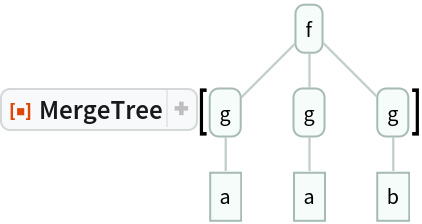Out=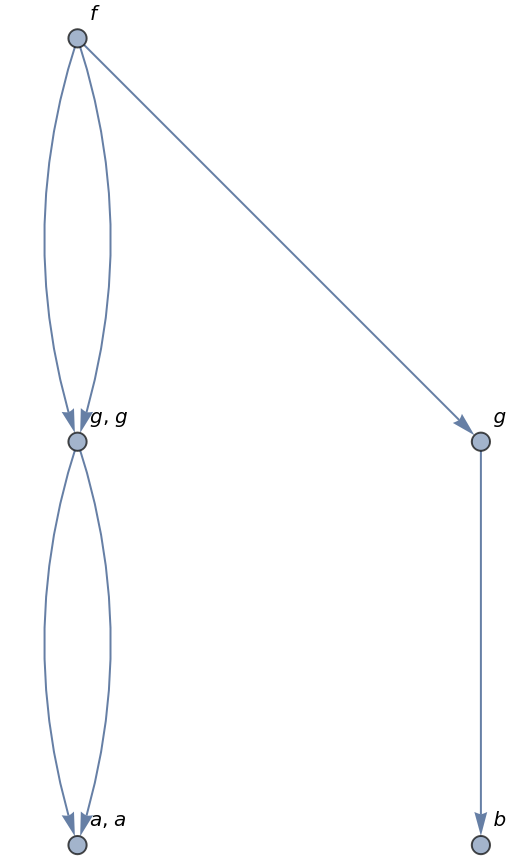Merge subtrees by a given function:

 In:=Out=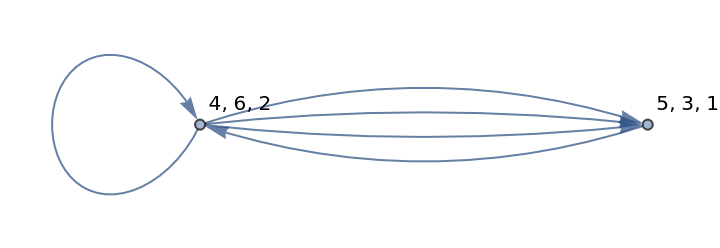### Scope (3)

Use TreeFold to merge subtrees after folding them:

 In:=Out=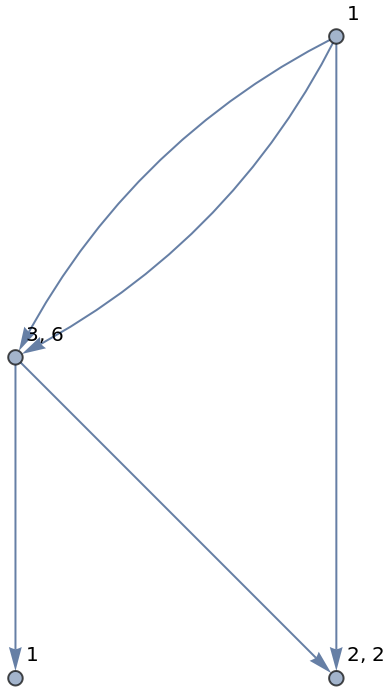Annotate a graph with edge tags showing original tree hierarchy:

 In:=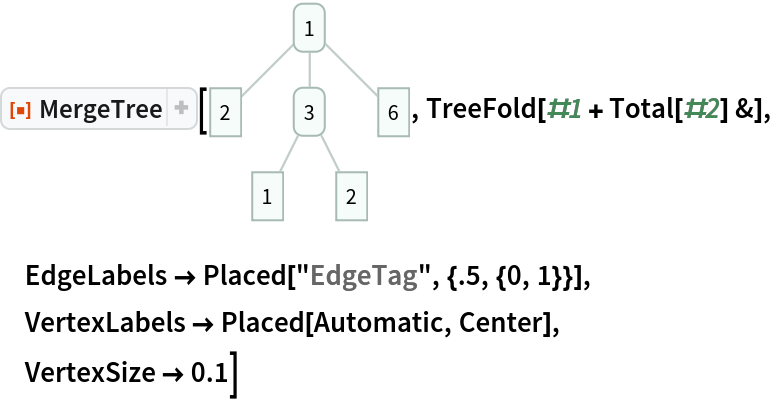Out=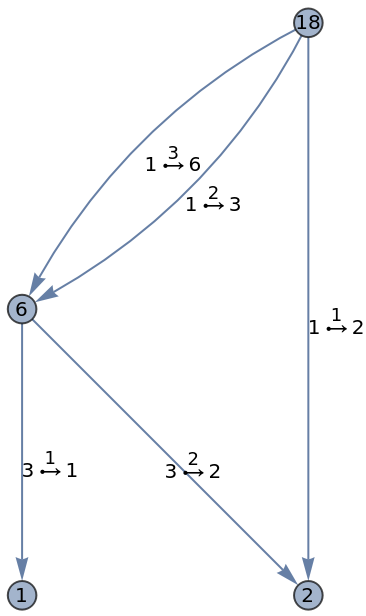Use the operator form of MergeTree:

 In:=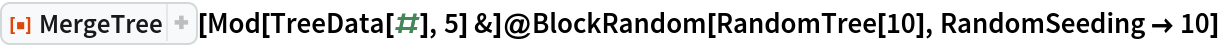Out=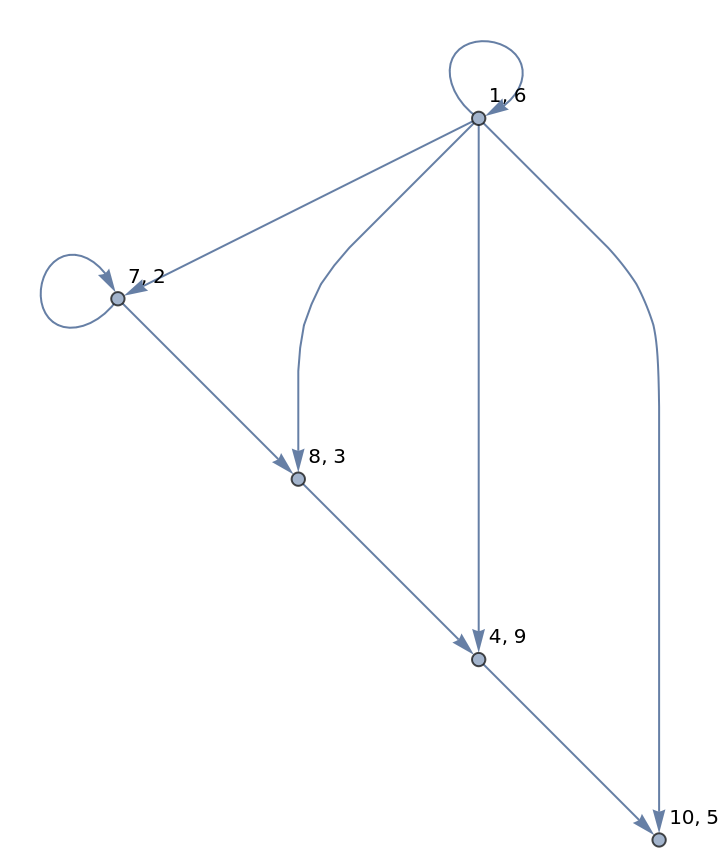### Options (1)

#### VertexLabelFunction (1)

Use a custom function to label vertices:

 In:=Out=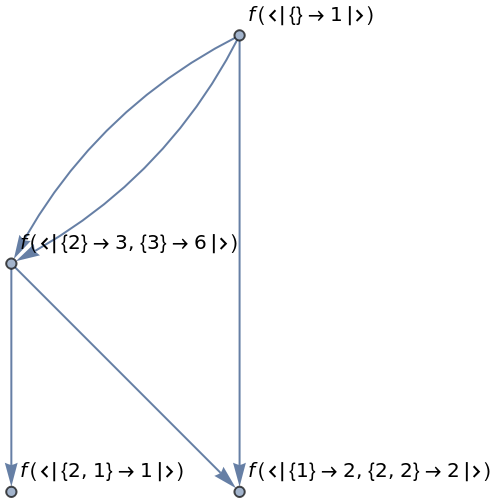### Applications (1)

Make a graph representation of AxiomaticTheory formulas:

 In:=Out=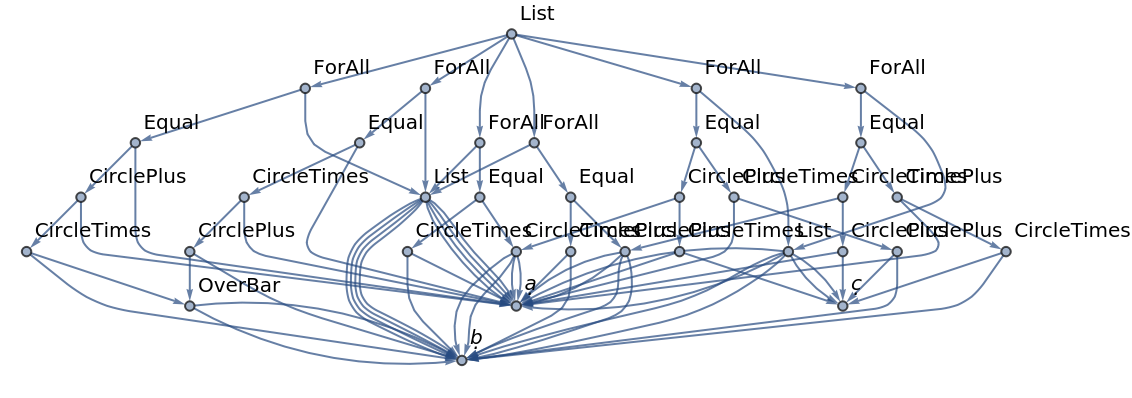## Version History

• 1.0.0 – 24 January 2022